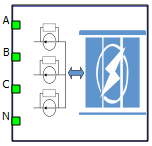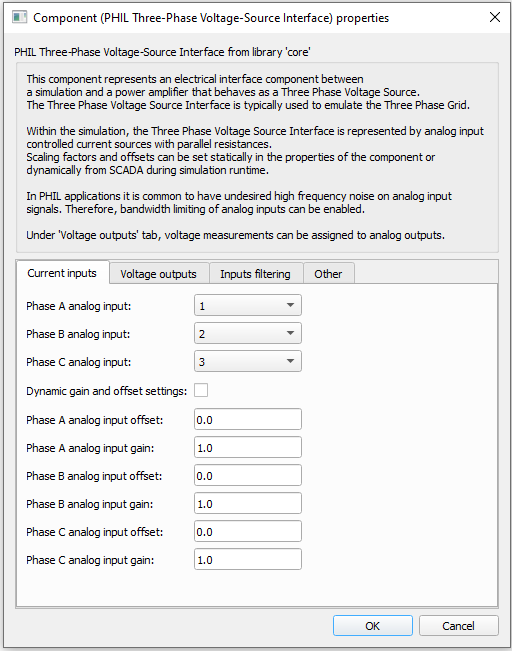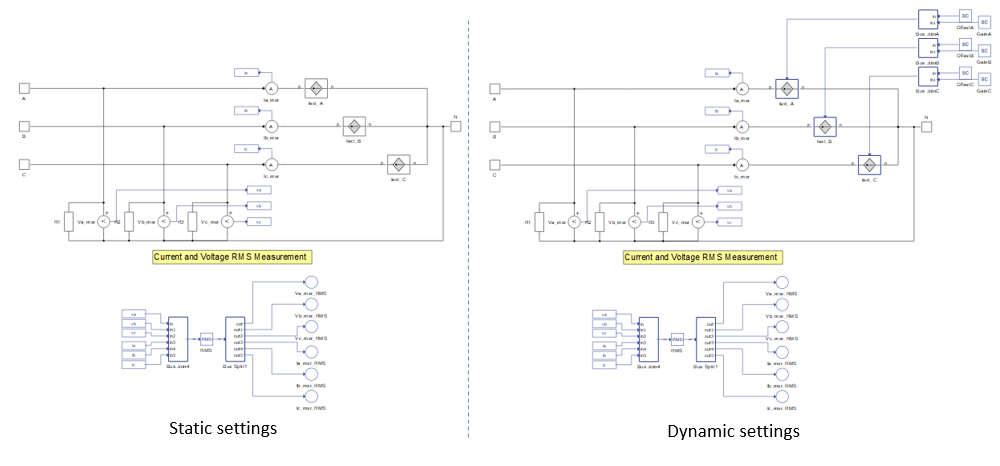# PHIL Three Phase Voltage Source Interface

This section describes the PHIL Three Phase Voltage Source Interface component, one of a collection of PHIL components for specific system applications. The PHIL setup where this interface component is used is a high power three-phase voltage-source.

The PHIL Three Phase Voltage Source is a PHIL interface component used to link the real time simulation with a real power amplifier, to form a PHIL system, by means of analog input/output signals. The PHIL Three Phase Voltage Source Interface component's internal structure is shown in Figure 1. It is composed of three analog input controlled current sources, voltage and current measurements, and a RMS calculation unit. As can be seen electrically within the simulated model, this interface component is represented by three current sources with parallel resistances. These current sources are defined through the analog inputs of the real-time simulator. These analog signals must be provided by the power amplifier. The analog signals that are sent to the power amplifier are the Va_msr, Vb_msr and Vc_msr voltages. These voltages are the references for the power amplifier, therefore the amplifier behaves as a three phase voltage source.

Table 1. PHIL Three Phase Voltage Source Interface in HIL Toolbox
component component dialog window component parametersPHIL Three Phase Voltage Source• Dynamic gain and offset settings
• Bandwidth enable
• Bandwidth limit
• Execution rate
• Series resistance

Dynamic gain and offset settings are available only if the selected real-time simulation HIL device supports Time Varying Elements. When the dynamic gain and offset is enabled, related SCADA inputs are added. This is shown in Figure 1.Figure 1. Schematic diagram of PHIL Three Phase Voltage Source Interface component

The list of measurements available in the PHIL Three Phase Voltage Source Interface is shown in table below.

## Internal analog measurements of the PHIL Three Phase Voltage Source Interface

Analog output variable name Description
(name).Ia_msr Instantaneous phase A current - usually used as a reference for the power amplifier.
(name).Ib_msr Instantaneous phase B current - usually used as a reference for the power amplifier.
(name).Ic_msr Instantaneous phase C current - usually used as a reference for the power amplifier.
(name).Va_msr Instantaneous phase A voltage.
(name).Vb_msr Instantaneous phase B voltage.
(name).Vc_msr Instantaneous phaseC voltage.
(name).Ia_msr_RMS Phase A RMS current.
(name).Ib_msr_RMS Phase B RMS current.
(name).Ic_msr_RMS Phase C RMS current.
(name).Va_msr_RMS Phase A RMS voltage.
(name).Vb_msr_RMS Phase B RMS voltage.
(name).Vc_msr_RMS Phase C RMS voltage.

A typical use case of the PHIL Three Phase Voltage Source Interface is in a Three Phase Grid Emulator PHIL system (Figure 2). The grid emulator defines the voltages for the high power device under test, therefore the power amplifier behaves as a voltage source. The three phase grid is simulated by the real-time simulation. The grid voltages are measured and fed to the power amplifier's analog inputs through the real-time simulator’s analog outputs. Currents measured by the power amplifier on its high power terminals are provided to the real-time simulation through analog inputs.